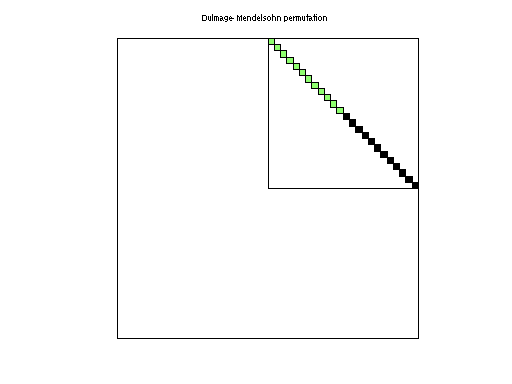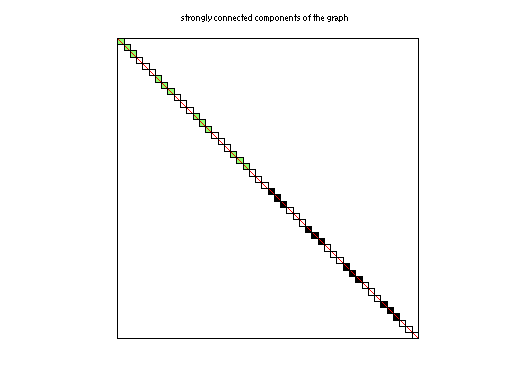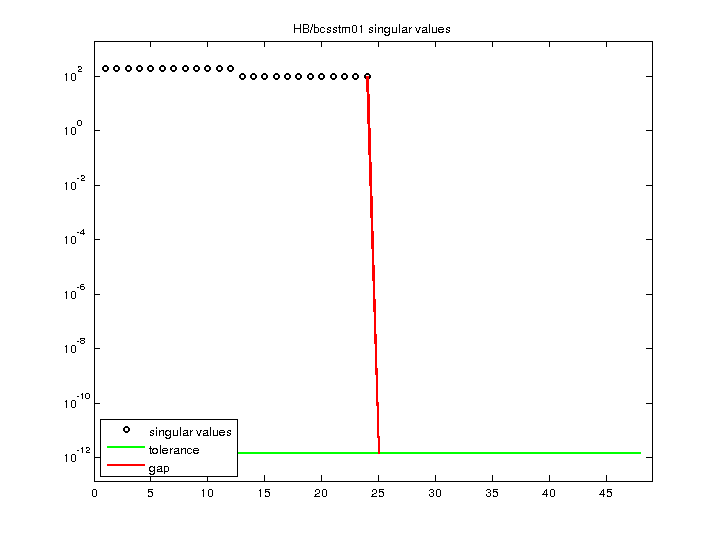Matrix: HB/bcsstm01

Description: SYMMETRIC MASS MATRIX SMALL GENERALIZED EIGENVALUE PROBLEM, B MATRIX(undirected graph drawing)• Matrix group: HB
• download as a MATLAB mat-file, file size: 621 bytes. Use UFget(56) or UFget('HB/bcsstm01') in MATLAB.

 Matrix properties number of rows 48 number of columns 48 nonzeros 24 structural full rank? no structural rank 24 # of blocks from dmperm 26 # strongly connected comp. 48 explicit zero entries 24 nonzero pattern symmetry symmetric numeric value symmetry symmetric type integer structure symmetric Cholesky candidate? no positive definite? no

 author J. Lewis editor I. Duff, R. Grimes, J. Lewis date 1982 kind structural problem 2D/3D problem? yes

 Ordering statistics: result nnz(chol(P*(A+A'+s*I)*P')) with AMD 48 Cholesky flop count 4.8e+01 nnz(L+U), no partial pivoting, with AMD 48 nnz(V) for QR, upper bound nnz(L) for LU, with COLAMD 48 nnz(R) for QR, upper bound nnz(U) for LU, with COLAMD 48

Note that all matrix statistics (except nonzero pattern symmetry) exclude the 24 explicit zero entries.

 SVD-based statistics: norm(A) 200 min(svd(A)) 0 cond(A) Inf rank(A) 24 sprank(A)-rank(A) 0 null space dimension 24 full numerical rank? no singular value gap Inf

 singular values (MAT file): click here SVD method used: s = sort (abs(full(diag(A))),'descend') ; since A is diagonal status: ok